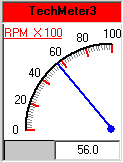CI MeterX > ActiveX Control Reference > Examples > Using VB Code to Set Properties at Runtime

#### Using VB Code to Set Properties at Runtime

This example shows how to configure a 90-degree dial meter with clockwise rotation using the VB codes. To try this example, add a MeterX control named TechMeter3 to a VB form, and paste the following code into the form's module:

Private Sub Form_Load()

With TechMeter3

.AlarmFlashColor = &HFFFF'Yellow

.AlarmFlashEnabled = False

.AlarmLowerBoundary = 50

.AlarmUpperBoundary = 80

.BackColor = &HFFFFFF       'White

.BorderStyle = [Fixed Single]

.Caption = "TechMeter3"

.CaptionAlignment = 2                 'vbCenter

.CaptionBackColor = &HFF'Red

With .CaptionFont

.Bold = True

.Name = "MS Sans Serif"

.Size = 8

End With

.CaptionForeColor = &H0      'Black

.CaptionVisible = True

.DegreeStart = 90

.DegreeEnd = 180

.Enabled = True

.EngUnit ="RPM  X 100"

.EngUnitColor = &HFF&       'Red

With .EngUnitFont

.Bold = True

.Name = "MS Sans Serif"

.Size = 8

End With

.EngUnitPosition = [Top Left] '0

.ForeColor = &H0          'Black

.Height = 2415  'Twip

.LabelColor = &H0        'Black

With .LabelFont

.Bold = True

.Name = "MS Sans Serif"

.Size = 8

End With

.LabelOffset = 22

.LabelOutside = True

.MajorDivColor = &HFF&      'Red

.ManualAdjustment = False

.MaxValue
= 100

.MeterTipText = ""

.MinorDivColor = &H808080  'Dark grey

.MinValue
= 0

.NeedleColor = &HFF0000   'Blue

.NeedleLength = 908  'Ignored as ManualAdjustment = false

.NeedleWidth = 2

.NumMajorDiv = 5

.NumMinorDiv = 10

With .ReadingFont

.Bold = True

.Name = "MS Sans Serif"

.Size = 8

End With

.RotateCenter = [Bottom Right] '4

.RotateDirection = [Anti Clockwise'1

.ScaleColor = &H0       'Black

.Value = 56

.ValueFormat = [#.#'1

.Width = 1815

.Xmid = 1615    'Ignored as ManualAdjustment = false

.Ymid = 1900    'Ignored as ManualAdjustment = false

End With

End Sub

This final result is shown as below.See Also: